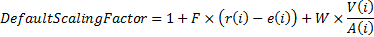/

### Function Description

Returns an array showing the probability of sponsor default over any given year in a pension fund projection.

If FactorDefaultAdjParam and WindUpDefaultAdjParam are both zero then the computations are very straightforward, depending only on BaseSponsorDefaultRates.

However, if FactorDefaultAdjParam or WindUpDefaultAdjParam are non-zero then the probability of default is adjusted from the above in a manner that reflecting the progression of the FactorDrivingDefault or by how well funded on a discontinuance basis the scheme is projected to be at future points in time, by applying a scaling factor to BaseSponsorDefaultRates (min 0, max 1) defined as follows:where: i = year in projection, F = FactorDefaultAdjParam, r(i) = Actual return (movement) on Factor Driving Default(i), e(i) = Expected return on Factor Driving Default(i), W = WindUpDefaultAdjParam, V(i) = TotalBenefitValue(i) (on a windup basis), A(i) = Total Asset Value(i)

(values of r(i), e(i), V(i) and A(i) are computed using Nematrian’s approximate pension projection algorithms)

Contents | Prev | Next